# Year 2 programme of study

Number - number and place value

Pupils should be taught to:

• count in steps of 2, 3, and 5 from 0, and in 10s from any number, forward and backward
• recognise the place value of each digit in a two-digit number (10s, 1s)
• identify, represent and estimate numbers using different representations, including the number line
• compare and order numbers from 0 up to 100; use <, > and = signs
• read and write numbers to at least 100 in numerals and in words
• use place value and number facts to solve problems

Pupils should be taught to:

• solve problems with addition and subtraction:
• using concrete objects and pictorial representations, including those involving numbers, quantities and measures
• applying their increasing knowledge of mental and written methods
• recall and use addition and subtraction facts to 20 fluently, and derive and use related facts up to 100
• add and subtract numbers using concrete objects, pictorial representations, and mentally, including:
• a two-digit number and 1s
• a two-digit number and 10s
• 2 two-digit numbers
• show that addition of 2 numbers can be done in any order (commutative) and subtraction of 1 number from another cannot
• recognise and use the inverse relationship between addition and subtraction and use this to check calculations and solve missing number problems

Number - multiplication and division

Pupils should be taught to:

• recall and use multiplication and division facts for the 2, 5 and 10 multiplication tables, including recognising odd and even numbers
• calculate mathematical statements for multiplication and division within the multiplication tables and write them using the multiplication (×), division (÷) and equals (=) signs
• show that multiplication of 2 numbers can be done in any order (commutative) and division of 1 number by another cannot
• solve problems involving multiplication and division, using materials, arrays, repeated addition, mental methods, and multiplication and division facts, including problems in contexts

Number - fractions

Pupils should be taught to:

• recognise, find, name and write fractions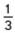,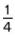,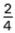and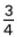of a length, shape, set of objects or quantity
• write simple fractions, for example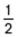of 6 = 3 and recognise the equivalence ofandMeasurement

Pupils should be taught to:

• choose and use appropriate standard units to estimate and measure length/height in any direction (m/cm); mass (kg/g); temperature (°C); capacity (litres/ml) to the nearest appropriate unit, using rulers, scales, thermometers and measuring vessels
• compare and order lengths, mass, volume/capacity and record the results using >, < and =
• recognise and use symbols for pounds (£) and pence (p); combine amounts to make a particular value
• find different combinations of coins that equal the same amounts of money
• solve simple problems in a practical context involving addition and subtraction of money of the same unit, including giving change
• compare and sequence intervals of time
• tell and write the time to five minutes, including quarter past/to the hour and draw the hands on a clock face to show these times
• know the number of minutes in an hour and the number of hours in a day.

Geometry - properties of shapes

Pupils should be taught to:

• identify and describe the properties of 2-D shapes, including the number of sides, and line symmetry in a vertical line
• identify and describe the properties of 3-D shapes, including the number of edges, vertices and faces
• identify 2-D shapes on the surface of 3-D shapes, [for example, a circle on a cylinder and a triangle on a pyramid]
• compare and sort common 2-D and 3-D shapes and everyday objects

Geometry - position and direction

Pupils should be taught to:

• order and arrange combinations of mathematical objects in patterns and sequences
• use mathematical vocabulary to describe position, direction and movement, including movement in a straight line and distinguishing between rotation as a turn and in terms of right angles for quarter, half and three-quarter turns (clockwise and anti-clockwise)

Statistics

Pupils should be taught to:

• interpret and construct simple pictograms, tally charts, block diagrams and tables
• ask and answer simple questions by counting the number of objects in each category and sorting the categories by quantity